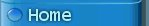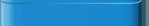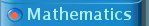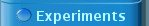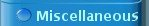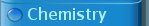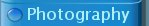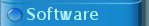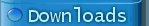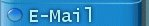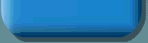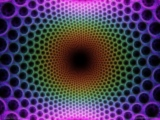### Mathematics: the Language of Science

Already in ancient times, long before the birth of Christ, people used special symbols and drawings for describing phenomena, which were hard to describe in words. Quantities of cattle, motion of celestial bodies, bookkeeping of amounts of silver and gold, all those things needed some notation which allowed people to describe them with great accuracy. Words were too inaccurate or too wieldy.

Both this practical need and the philosophy of ancient thinkers lead to the first steps into mathematics. The ancient Greeks were capable of solving quadratic equations, they had a notion of the number π and they had found all kinds of ingenious constructions for geometrical patterns, using a straight stick and compass only.

With the advent of modern science at the end of the middle ages and the start of Renaissance, mathematics also became more and more important. In the 16th century, study of kinematics lead to the concepts of velocity and acceleration. Describing these concepts in a quantitative way is very hard without a rigorous notation and where Galileo still lacked suitable tools for describing these concepts in a general way, Newton introduced new concepts, such as rate of change of a quantity, the associated infinitesimals and the use of variables as a notation for specific quantities. In this way, the foundations were laid of the core concepts of calculus. Newton only used these concepts in the domain of mechanics, but soon it became clear that these concepts were very useful in describing many other phenomena and solving numerous problems in other domains of physics.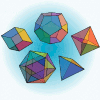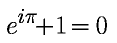Since then, more and more use is made of mathematics. Physics now is unimaginable without mathematics. Physics has been a strong catalyst for developing new mathematical concepts over the past three centuries. Nice examples are:

• Differential equations for describing the change of quantities, such as velocity of an object under the influence of a force.
• The concepts of vector fields and the associated vector calculus when theories were developed about electromagnetism.
• Tensor calculus and differential geometry, needed for describing general relativity.
• Concepts like Fourier transforms, Laplace transforms, frequency domain and time domain, used in signal processing and linear control theory. This theory was developed at the start of the 20th century with the introduction of electronic devices.

One can safely state that mathematics has made possible modern physics. Without mathematics there could not be physics as we know it nowadays.

At a certain point in time, mathematics has become a science on its own. There are many mathematical concepts, which do not come from a problem in physics, and nowadays mathematics opens up a whole new bunch of applications such as cryptography, used in secure communications, or even artwork, in which mathematics is used for rendering virtual worlds.

In this webpage, different aspects of mathematics are emphasized, not simply by theory, but in the form of experiments, which can be carried out by others and which demonstrate the concepts behind the mathematics. It is believed and hoped that this may add to the fascination for mathematics and for science in general.